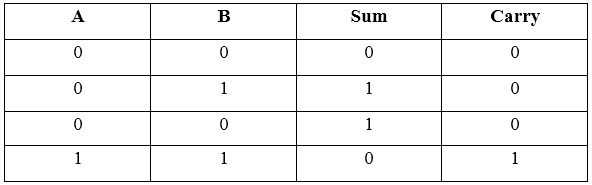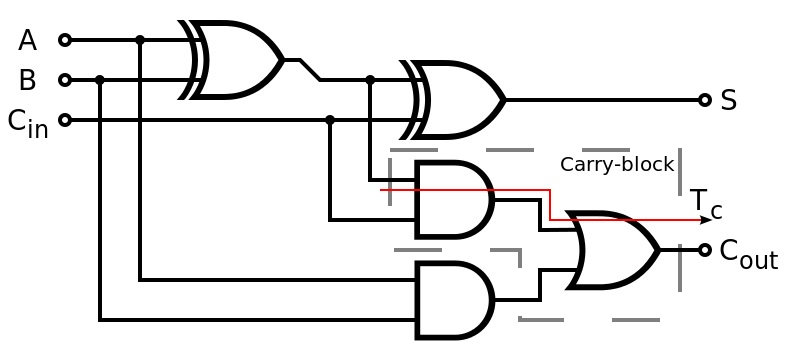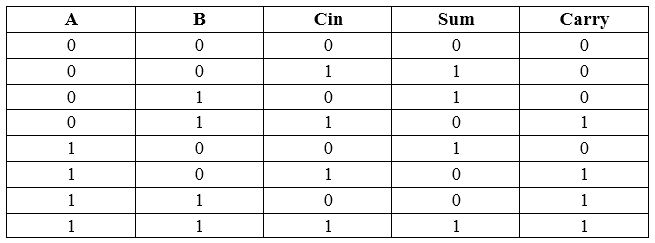# What is the Difference Between Half Adder and Full Adder Circuit

The main difference between half adder and full adder circuit is that half adder circuit performs an addition of two 1- bit numbers while full adder circuit performs the addition of three 1- bit numbers.

Digital Circuit is a circuit that consists of logic gates to represent Boolean logic functions. One type of digital circuit is a combinational logic circuit. The output of these circuits depends on the present inputs. These circuits do not have memory elements. An adder is a combinational circuit that is capable of performing arithmetic additions on binary data. There are two adder circuits as a half adder and full adder. Both these circuits provide sum and carry as the output.

### Key Areas Covered

1. What is Half Adder Circuit
– Definition, Functionality
2. What is Full Adder Circuit
– Definition, Functionality
3. What is the Difference Between Half Adder and Full Adder Circuit
– Comparison of Key Differences

### Key Terms## What is Half Adder Circuit

A half adder is a circuit that is capable of adding two 1 bit numbers. It has two 1 bit inputs and two outputs.

The circuit diagram is as follows.The truth table is as follows. A and B are the inputs whereas Sum and Carry are the outputs.Boolean expression for sum is S = A B / + A / B

Boolean expression for carry is S = A B

A full adder is a circuit that is capable of adding three 1-bit numbers. In addition to two 1 bit inputs, there is another input which is generated from the previous bit addition.

The circuit diagram is as follows.The truth table is as follows. A and B are the two 1 bit inputs. Cin is a carry bit generated from previous addition. Thus, there are altogether three inputs. Sum and Carry are the outputs.Boolean expression for sum is S = A / B / Cin + A / B C/ + A B Cin + A B / Cin /

Boolean expression for carry is S = A B + A Cin + B Cin

It is possible to represent a full adder using two half adders.

### Definition

A half adder is an adder circuit that adds two single binary digits and provides the sum and the carry bit while Full adder is an adder circuit that adds three one bit binary numbers that provide the sum and a carry bit. This is the main difference between half adder and full adder circuit.

### Input

Moreover, half adder has two operands as the input (A, B) while full adder has two operands (A, B) and carry bit (Cin) as the input.

### Previous Carry Bit

In half adder, there is no previous carry bit as an input. However, in full adder, there is a previous carry bit as an input. This is another difference between half adder and full adder circuit.

### Conclusion

##### Reference:

1. Kharate, G K. Digital Electronics. Oxford University Press, 2010
2. “What Is a Half Adder? – Definition from Techopedia.” Techopedia.com, Available here.
3. “What Is a Full Adder? – Definition from Techopedia.” Techopedia.com, Available here.

##### Image Courtesy:

1. “Half Adder” By inductiveload – Own work (Public Domain) via Commons Wikimedia
2. “Full-adder logic diagram” By Inductiveload – Own work (Public Domain) via Commons Wikimedia